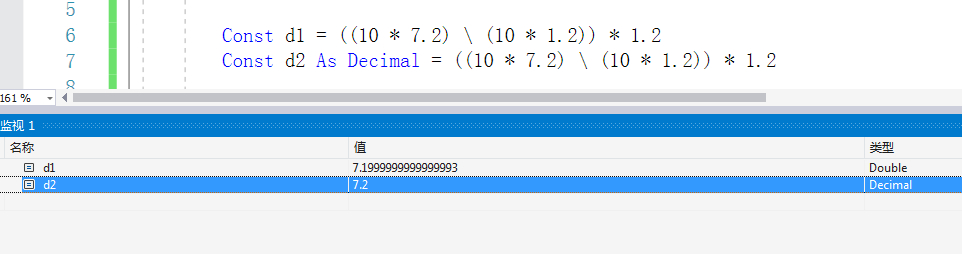•闪存
•博客
• 发言小组
• 投递新闻
• 提问博问
• 添加收藏
• 发布招聘
•文库

# if语句输出问题

0悬赏园豆：5 [已解决问题] 解决于 2021-02-19 16:49If ((10 * 7.2) \ (10 * 1.2)) * 1.2 = 7.2 Then
MsgBox(((10 * 7.2) \ (10 * 1.2)) * 1.2)
MsgBox("true")
Else
MsgBox(((10 * 7.2) \ (10 * 1.2)) * 1.2)
MsgBox("false")
End If

1

vb默认精度问题。

((10 * 7.2) \ (10 * 1.2)) * 1.2 计算出来的值并不是7.2，而是7.19999……leslie_xin | 菜鸟二级 |园豆：358 | 2021-02-19 16:19

Dim a As Decimal = ((10 * 7.2) \ (10 * 1.2)) * 1.2
If a = 7.2 Then
MsgBox("true")
Else
MsgBox("false")
End If

LFMB | 园豆：158 (初学一级) | 2021-02-19 16:45

0

您需要登录以后才能回答，未注册用户请先注册